# The Formula Column

Missing Excel formulas? Not to worry! We came up with the Formula Column just for you. It's capable of nearly any calculation from simple mathematical equations to more complex formulas. With our functions, you can create standalone calculations or pull data in from other columns to give you the information you need. 🙌Note:  The Formula Column is only available for the Pro and Enterprise plan. If you were on a Pro plan and downgraded your account, the formula columns you have created will remain intact, but you will not be able to add more formula columns.

To add it, click on the + icon located on the top right of your board and select "Formula" from the resulting dropdown menu: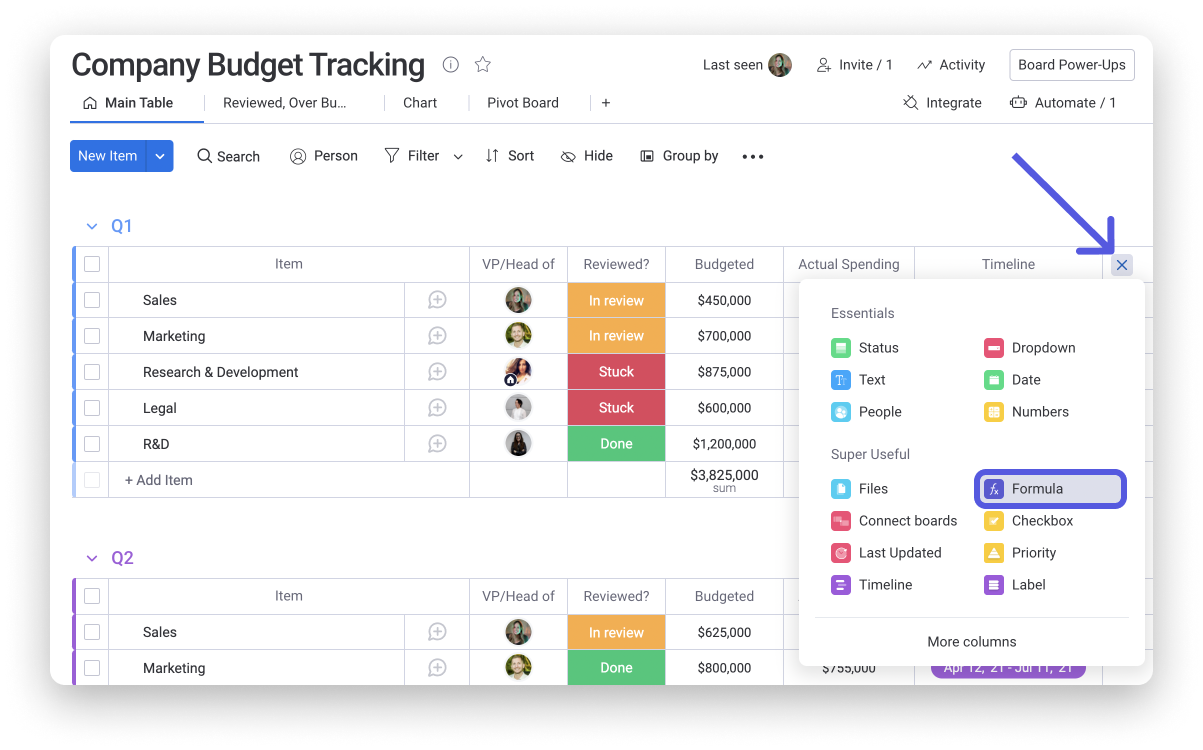# How it works

The formula builder will automatically open when you add the Formula Column. If you've exited the formula builder, simply click anywhere within the column to begin building your formula.

In the formula builder, you'll first find a list of the columns on your board which you can use within the calculations that you create, followed by the list of available functions that we offer. To build a formula, you can use any of the available functions or you can use traditional math symbols.If you hover over a function, the display will show you a description of the function and an example of how it works:To add a formula, click into a cell within the Formula Column and begin to type out your formula. Here, you can use the columns from your board as well a variety of functions to build out the formula that you need!Let's take our Company Budget Tracking board for example. Here, we want to easily show if our actual spending is over or under the budget that was allotted to it. We used the below formula to see this information at a glance!Formula: IF({Budgeted}>{Actual Spending},"Over budget","Under budget")

# What do the colors signify?

You may have noticed that certain parts of your formula (functions, columns, punctuations) are colored within the editor! We refer to this as "syntax highlighting" and it allows you to build out your formula with ease, and to prevent or allow you to notice potential errors within your formula. Red coloring indicates an error in your formula.

Let's take the below formula for example, where we are attempting to subtract the "Actual Spending" column from the "Budgeted" column:Here, we can see that there is a red parenthesis at the end of our formula. The reason for this is that that parenthesis is redundant and not needed! As soon as we delete the final parenthesis, our formula will be good to go. 🙌Tip: Check out the "General rules" section below to learn how about how to prevent receiving errors in your formula!

# General rules

• Using brackets

When building a formula, you may need to reference a column in order to pull that column’s value into your function. To do that, you’ll need to include the name of the column you’re referencing within these symbols: {}

It is important to use the columns’ name exactly as it appears on your boards. For example, this column: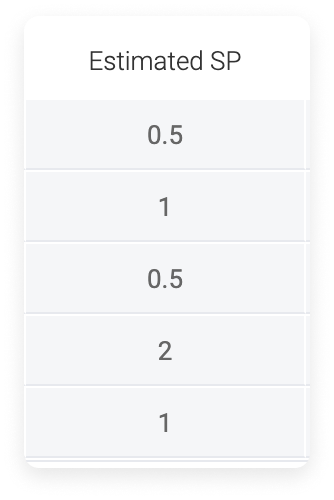Must be typed as “{Estimated SP}”. Like this:

`SUM({Estimated SP},10)`

This will take the value in the “Estimated SP” column and add 10 to it.

• Values are case sensitive

Treat values as if they’re case sensitive. So, if your column name is “Estimate SP”, be sure to use a capital E, a capital S, and capital P in your formula.

This is correct: `SUM({Estimated SP},10)`

This is incorrect: `SUM({estimate sp},10)`Note: This rule is especially true if you create a formula that references a Status label.

• The comma is dynamic

When used in the formula column, the comma symbol represents something different depending on the function. Take the above formula as an example. In this formula:

`SUM({Estimated SP},10)`

The comma represents an addition symbol, also known as +.

In the following function:

`MULTIPLY({Estimated SP},2)`

The comma represents a multiplication symbol, also known as x.

When using the IF function, the comma represents the separation of each component of a conditional statement. More on that later.

• Using parentheses

When you open a statement, you must also close the statement. You open a statement with a ( and close it with a ). For example:

``` MULTIPLY(SUM({Estimated SP},10),2) ```

The above formula will take the Sum of the Estimated SP column plus 10 and multiply that total by 2.

• Using quotation marks

When a statement reads text of any kind, whether a Status label or a Text Column, you must put the text in quotation marks. For example, this formula is looking for the word "Yes" in the Text Column:

`IF({Text}="Yes","Awesome!","Too bad.")`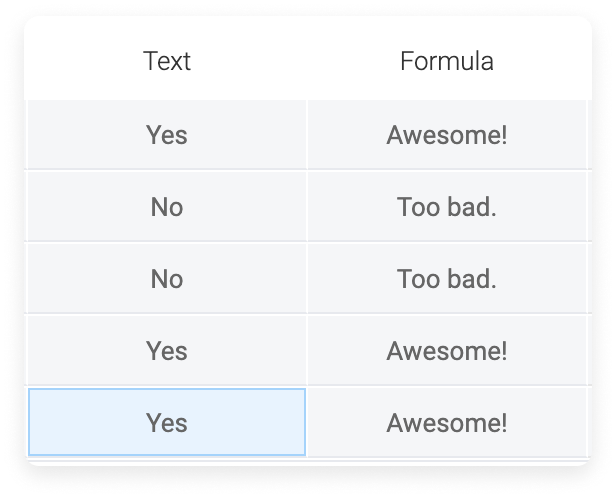Using a text editor to write your formula and then copying and pasting will result in the formula builder reading it as illegal. The formatting of quotation marks and commas can impact the effectiveness of your formula. Take a look at the quotation marks in the following.

This is correct: `IF({Text}="Yes","Awesome!","Too bad.")`

This is incorrect: `IF({Text}=”Yes”,”Awesome!”,”Too bad.”)`

• Using decimals

When multiplying by a decimal, always include a 0 before the decimal. If not, your formula will return an "Illegal Formula" error.

This is correct: `MULTIPLY(10,0.25)`

This is incorrect: `MULTIPLY(10,.25)`

# Compatible columns

Much like formulas in Excel, the Formula Column has its own logic. But not to worry! We're here to help you figure it out. The following are the compatible and incompatible columns.Show me the compatible columns

Compatible columns

• Check, Country, Creation Log, Date, Dependency, Dropdown, Email, Formula, Hour, Item ID, Last Updated, Connect Boards (previously Link to Item), Long Text, Numbers, Person, Phone, Rating, Status, Text, Timeline, Time Tracking, Vote, World Clock
• Subitem Names and the Count of Subitems are also supported.

Incompatible columns

• Autonumber, Color Picker, Files, Link, Location, Mirror, Progress Tracking, Tags, Week

# Limitations

1. Any formula you add will apply to the entire column.

2. The Formula Column will read the board horizontally, meaning that it can not execute calculations vertically.

3. The Formula Column can read data from other column cells, but not from the column summary or any other area of the board.

4. It also can not read other boards, so a function like a VLOOKUP is not possible at this time.

# The IF function

The IF function is one of the most commonly used formula types and it is used to create a conditional statement. If you aren’t familiar with conditional statements, we can help! The IF statement will return or output one of two values. Let’s use this as an example: IF(a=true,b,c)

Or put in simpler terms:

If a is true, then return b.

If a is false, then return c.

The result, whether the formula returns a value of b or c, is conditional upon whether or not a is true.

Let’s look at that using a board and the Formula Column. For this example, we want the Formula Column to read “YAY!” when a task is done. If the task isn’t done, we want the formula to say “Not yet…”. To achieve this, we would use this formula:

`IF({Status}="Done","YAY!","Not yet...")`

Take a look at the results of this formula: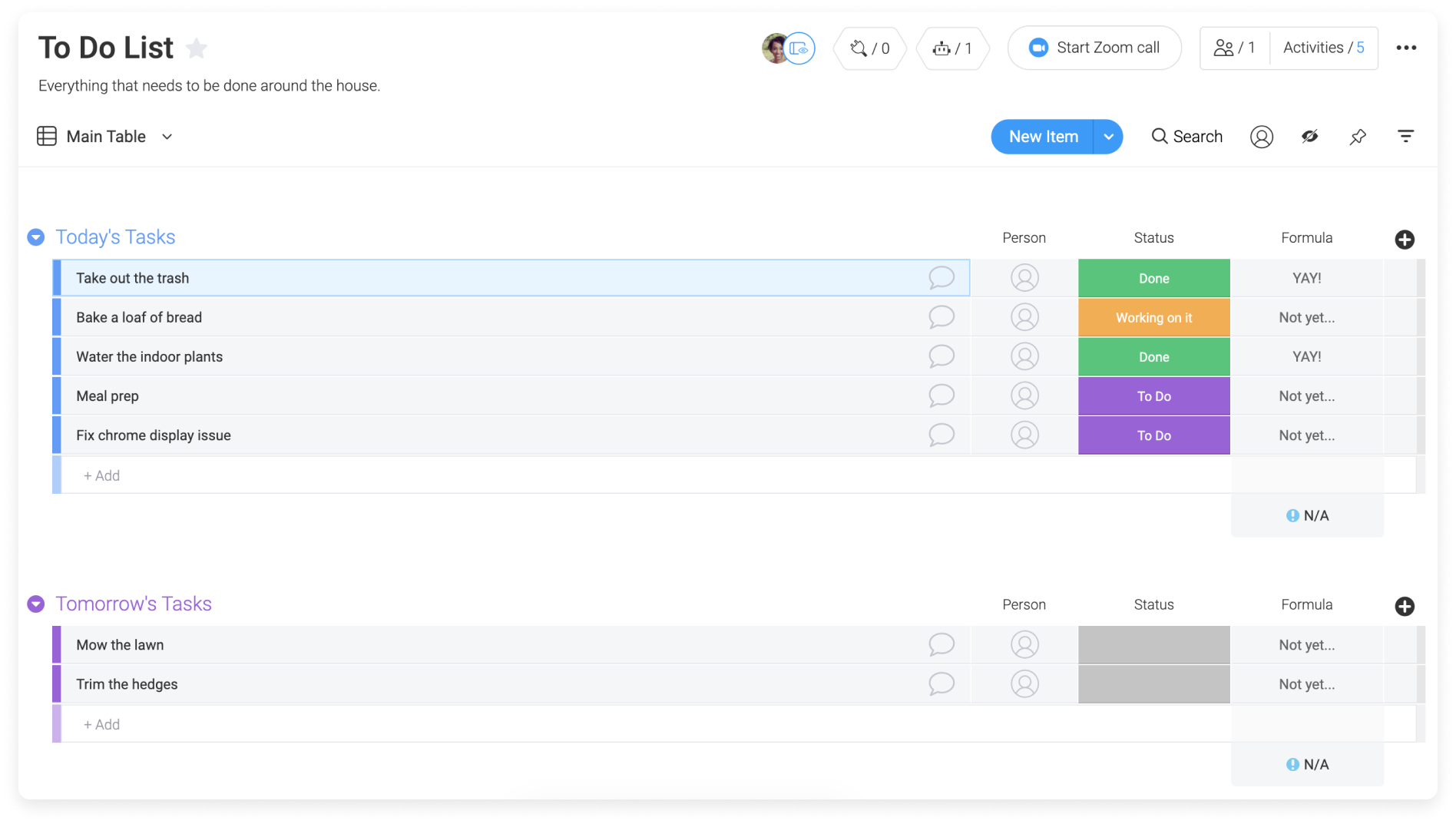You'll notice in the above results, that any label that isn't "Done" reads as "Not yet...". If I want to add to the IF statement so that the "Working on it" label outputs "Almost." in the Formula Column, I'll need to build from my original statement.

`IF({Status}="Done","YAY!","Not yet...")`

becomes

``` IF({Status}="Done","YAY!",IF({Status}="Working on it","Almost.","Not yet...")) ```

That means: IF(a=true,b,IF(c=true,d,e))

Or put in simpler terms:

If a is true, then return b.

If c is true, then return d.

If a and c are false, then return e.

You can build on this nesting of IF() statements endlessly. But remember! Anytime you open a parenthesis, you must close it. See the colorful parenthesis in the formula above for an example of how this works.# Customization

Just as with the Numbers Column, you can see the overall calculation for each group. You will also be able to change the unit and the function as below: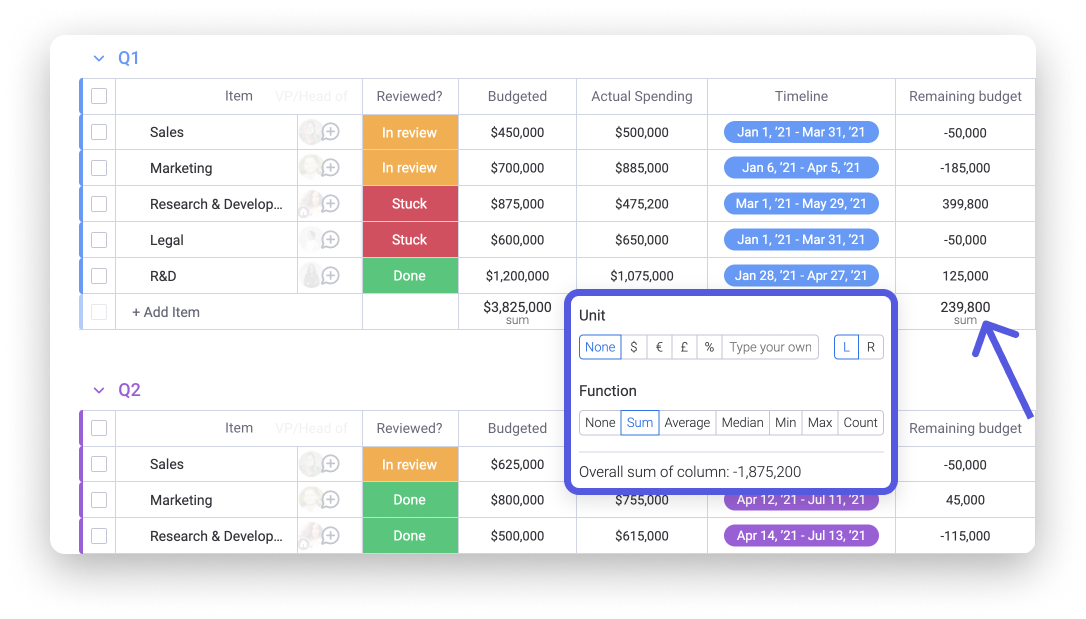Note: The total only takes into account numeric values. If your column has both numeric and text values, the summary will only count the numeric values. Mixed values like Lea123 will not be taken into account in the total. If all values are non-numeric, your summary will return N/A.

# Use cases

The possibilities with the Formula Column are endless! To get an idea of how the Formula Column can work for you, check out our article on Formula Use Cases as well as the board below.

For a list of all of the available functions, check out our Formula Library.

If you have any questions, please reach out to our team right here. We're available 24/7 and happy to help.